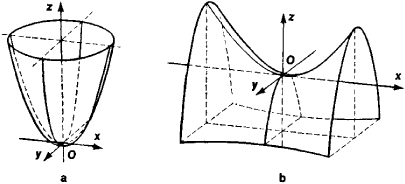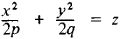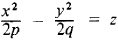# paraboloid

(redirected from Circular paraboloid)
Also found in: Dictionary, Thesaurus.
Related to Circular paraboloid: hyperbolic paraboloid, Paraboloid of revolution

## paraboloid

(pă-rab -ŏ-loid) A curved surface formed by the rotation of a parabola about its axis. Cross sections along the central axis are circular. A beam of radiation striking such a surface parallel to its axis is reflected to a single point on the axis (the focus), no matter how wide the aperture (see illustration). A paraboloid mirror is thus free of spherical aberration; it does however suffer from coma. Paraboloid surfaces are used in reflecting telescopes and radio telescopes. Over a small area a paraboloid differs only slightly from a sphere. A paraboloid mirror can therefore be made by deepening the center of a spherical mirror.

## Paraboloid

an open quadric surface without a center. There are two types of paraboloids—elliptic and hyperbolic (Figure 1). Paraboloids are two of the five main types of quadric surfaces. The intersection of a hyperbolic paraboloid with a plane isFigure 1. Paraboloids: (a) elliptic, (b) hyperbolic

a hyperbola, a parabola, or a pair of lines. Two rectilinear generators pass through each point of a hyperbolic paraboloid, which consequently is a ruled surface. In contrast to a hyperbolic paraboloid, an elliptic paraboloid does not intersect every plane in space. When it does intersect a plane, the intersection is either an ellipse or a parabola. In an appropriate system of coordinates the equation for an elliptic paraboloid has the formand the equation for a hyperbolic paraboloid has the formHere, p > 0 and q > 0.

## paraboloid

[pə′rab·ə‚lȯid]
(engineering)
A reflecting surface which is a paraboloid of revolution and is used as a reflector for sound waves and microwave radiation.
(mathematics)
A surface where sections through one of its axes are ellipses or hyperbolas, and sections through the other are parabolas.
Site: Follow: Share:
Open / Close